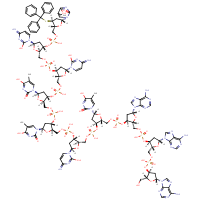Structure InformationCompound Identification

SMILES

CC1=CN([C@H]2C[C@H](OP(O)(=O)OC[C@H]3O[C@H](C[C@@H]3OP(O)(=O)OC[C@H]3O[C@H](C[C@@H]3OP(O)(=O)OC[C@H]3O[C@H](C[C@@H]3OP(O)(=O)OC[C@H]3O[C@H](C[C@@H]3OP(O)(=O)OC[C@H]3O[C@H](C[C@@H]3OP(O)(=O)OC[C@H]3O[C@H](C[C@@H]3SC(C3=CC=CC=C3)(C3=CC=CC=C3)C3=CC=CC=C3)N3C=C(C)C(=O)NC3=O)N3C=CC(N)=NC3=O)N3C=CC(N)=NC3=O)N3C=C(C)C(=O)NC3=O)N3C=C(C)C(=O)NC3=O)N3C=CC(N)=NC3=O)[C@@H](COP(O)(=O)O[C@H]3C[C@@H](O[C@@H]3COP(O)(=O)O[C@H]3C[C@@H](O[C@@H]3COP(O)(=O)O[C@H]3C[C@@H](O[C@@H]3CO)N3C=NC4=C3N=CN=C4N)N3C=NC4=C3N=CN=C4N)N3C=NC4=C3N=CN=C4N)O2)C(=O)NC1=O

InChIKey

InChIKey=KWOODUBBFULVJU-CQCVISGTSA-N

Formula

C116H139N32O58P9S

Mass

3220.38

### Export to:

Taxonomic Classification

Taxonomy Tree

Kingdom

Organic compounds

Superclass

Nucleosides, nucleotides, and analogues

Class

Nucleoside and nucleotide analogues

Subclass

Oligonucleotides

Intermediate Tree Nodes

Not available

Direct Parent

Oligonucleotides

Alternative Parents

Molecular Framework

Aromatic heteropolycyclic compounds

Substituents

(3'->5')-oligonucleotide - Purine deoxyribonucleoside 3',5'-bisphosphate - Purine deoxyribonucleoside bisphosphate - Pyrimidine deoxyribonucleoside 3',5'-bisphosphate - Pyrimidine deoxyribonucleoside bisphosphate - Triphenyl compound - 2',3'-dideoxy-3'-thionucleoside monophosphate - Ribonucleoside 3'-phosphate - 2',3'-dideoxy-3'-thionucleoside - 6-aminopurine - Imidazopyrimidine - Purine - Aminopyrimidine - Pyrimidone - Dialkyl phosphate - Monocyclic benzene moiety - Hydropyrimidine - N-substituted imidazole - Organic phosphoric acid derivative - Phosphoric acid ester - Pyrimidine - Alkyl phosphate - Imidolactam - Benzenoid - Heteroaromatic compound - Imidazole - Oxolane - Vinylogous amide - Azole - Urea - Lactam - Organoheterocyclic compound - Azacycle - Oxacycle - Dialkylthioether - Sulfenyl compound - Thioether - Hydrocarbon derivative - Amine - Organic oxygen compound - Organic oxide - Organonitrogen compound - Organooxygen compound - Organosulfur compound - Primary alcohol - Organic nitrogen compound - Alcohol - Primary amine - Aromatic heteropolycyclic compound

Description

This compound belongs to the class of organic compounds known as oligonucleotides. These are organic polymers made up of a sequence of 3 to 12 purine or pyrimidine nucleotide residues linked to one another from the 5' -end to the 3'-end through a phosphate group.

External Descriptors

Not available